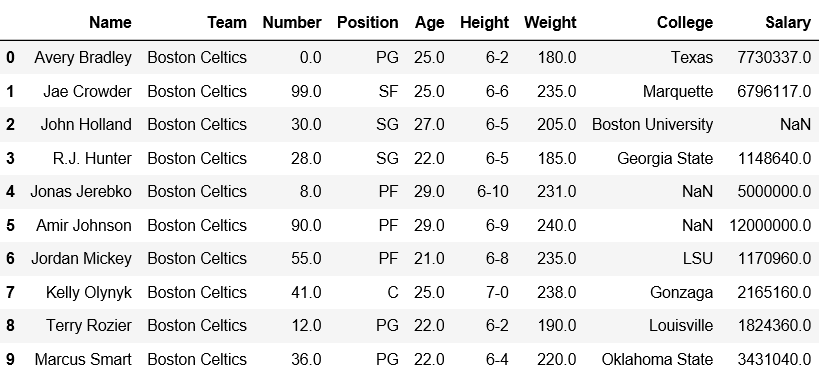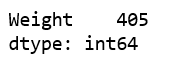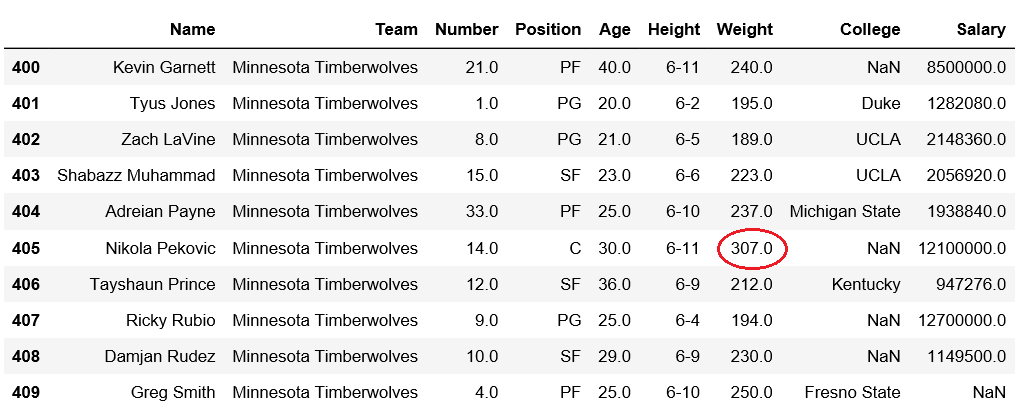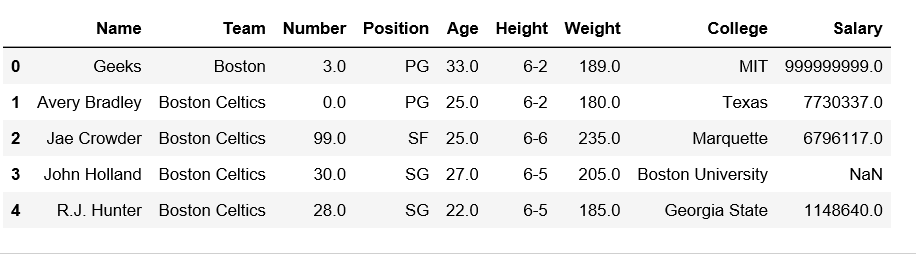GFG App
Open AppBrowser
Continue

# Get the index of maximum value in DataFrame column

Pandas DataFrame is two-dimensional size-mutable, potentially heterogeneous tabular data structure with labeled axes (rows and columns).
Let’s see how can we get the index of maximum value in DataFrame column.
Observe this dataset first. We’ll use ‘Weight’ and ‘Salary’ columns of this data in order to get the index of maximum values from a particular column in Pandas DataFrame.

## Python3

 `# importing pandas module ` `import` `pandas as pd ` `  `  `# making data frame ` `df ``=` `pd.read_csv(``"https://media.geeksforgeeks.org/wp-content/uploads/nba.csv"``) `   `df.head(``10``)`Code #1: Check the index at which maximum weight value is present.

## Python3

 `# importing pandas module ` `import` `pandas as pd ` `  `  `# making data frame ` `df ``=` `pd.read_csv(``"nba.csv"``)`   `# Returns index of maximum weight` `df[[``'Weight'``]].idxmax()`

Output:We can verify whether the maximum value is present in index or not.

## Python3

 `# importing pandas module ` `import` `pandas as pd ` `   `  `# making data frame ` `df ``=` `pd.read_csv(``"nba.csv"``)` ` `  `# from index 400 to 409` `df.iloc[``400``:``410``]`

Output:Code #2: Let’s insert a new row at index 0, having maximum salary and then verify.

## Python3

 `# importing pandas module ` `import` `pandas as pd ` `   `  `# making data frame ` `df ``=` `pd.read_csv(``"nba.csv"``)`   `new_row ``=` `pd.DataFrame({``'Name'``:``'Geeks'``, ``'Team'``:``'Boston'``, ``'Number'``:``3``,` `                        ``'Position'``:``'PG'``, ``'Age'``:``33``, ``'Height'``:``'6-2'``,` `                        ``'Weight'``:``189``, ``'College'``:``'MIT'``, ``'Salary'``:``999999999``}` `                         ``, index``=``[``0``])` ` `  `df ``=` `pd.concat([new_row, df]).reset_index(drop``=``True``)` `df.head(``5``)`

Output:Now, let’s check if the maximum salary is present at index 0 or not.

## Python3

 `# Returns index of minimum salary` `df[[``'Salary'``]].idxmax()`

Output:My Personal Notes arrow_drop_up